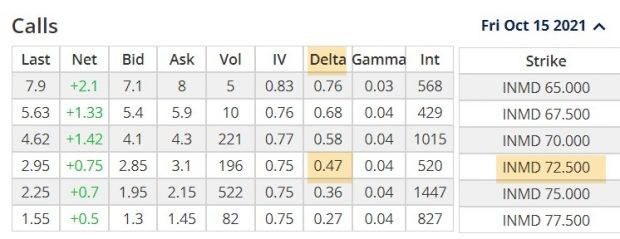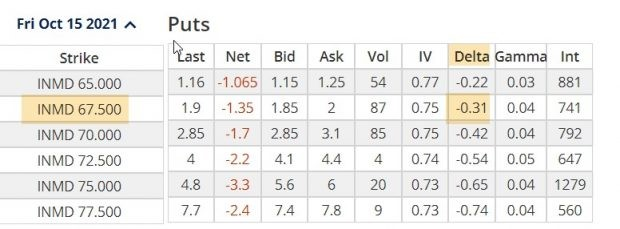Many portfolio managers will seek Delta-neutral portfolios to mitigate directional or market risk, states Allan Ellman of The Blue Collar Investor.

This article will explain how these experts calculate their positions to achieve this goal. To simplify the details, a single security will be used, but the concepts apply to total portfolio positions as well. Let's look at In Mode Ltd. (INMD).

Definitions

• Delta: The amount an option value will change for every \$1.00 change in the price of the underlying security.
• Gamma: The amount Delta will change for every \$1.00 change in the price of the underlying security.
• Delta-neutral: The sum of all put, call and stock Deltas are equal to or near zero.

Delta Ranges Per-Share

• Long stock: Always (+1)
• Short stock: Always (-1)
• Long calls: (0 to+1)
• Short calls: (0 to -1)
• Long puts: (0 to -1)
• Short puts: (0 to +1)
• For contracts multiply by 100

Delta-Neutral Goal

Use a combination of long and short calls and puts and stocks to create a portfolio with a net Delta position near zero.

***Gamma will be constantly changing Deltas. This concept is beyond the scope and goals of this article.

INMD (\$71.15) Call Delta for the OTM \$72.50 StrikeINMD Call Delta: 0.47

INMD (\$71.15) Put Delta for the OTM \$67.50 StrikeINMD Put Delta of 0.31

Delta-Neutral Calculations With Options Only

• We first establish a ratio of call Delta to put Delta: 0.47/0.31 = 1.5
• We will buy 1.5 times more puts than calls to reach a Delta-neutral position
• 4 calls = 4 x 0.47 = Delta of 1.88 x 100 = 188
• 6 puts = 6 x (-) 0.31 = Delta of – 1.86 x 100 = -186
• Net Delta position = +0.02 x 100 = +2 or near zero

Delta-Neutral Calculations With Stock and Options

• Buy 2 calls = +0.94 x 100 = 94
• Buy 4 puts = -.1.24 x 100 = -124
• Buy 30 shares = +1 x 30 = +30
• Net Delta position = zero or a true Delta-neutral position

Discussion

Although the concept of Delta-neutral portfolios generally does not apply to retail investors, there are times, during market turmoil, when we may look to achieve positions that are directionally protected. This article’s goal was to offer a methodology commonly used by professionals to manage these scenarios.